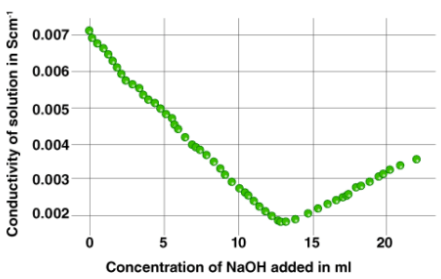# Conductometry & Conductometric Titrations Notes | Study Inorganic Chemistry - Chemistry

## Chemistry: Conductometry & Conductometric Titrations Notes | Study Inorganic Chemistry - Chemistry

The document Conductometry & Conductometric Titrations Notes | Study Inorganic Chemistry - Chemistry is a part of the Chemistry Course Inorganic Chemistry.
All you need of Chemistry at this link: Chemistry

What is Conductometric Titration?

Conductometric titration is a laboratory method of quantitative analysis used to identify the concentration of a given analyte in a mixture. Conductometric titration involves the continuous addition of a reactant to a reaction mixture and the documentation of the corresponding change in the electrolytic conductivity of the reaction mixture. It can be noted that the electrical conductivity of an electrolytic solution is dependent on the number of free ions in the solution and the charge corresponding to each of these ions.
In this type of titration, upon the continuous addition of the titrant (and the continuous recording of the corresponding change in electrolytic conductivity), a sudden change in the conductivity implies that the stoichiometric point has been reached. The increase or decrease in the electrolytic conductivity in the conductometric titration process is linked to the change in the concentration of the hydroxyl and hydrogen ions (which are the two most conducting ions).
The strength of an acid can be determined via conductometric titration with a standard solution of a base. An example of a curve plotted for such a titration process is given below.The method of conductometric titration is very useful in the titration of homogeneous suspensions or coloured solutions as these titrations cannot be done with the use of normal chemical indicators.

Principle

The principle of the conductometric titration process can be stated as follows – During a titration process, one ion is replaced with another and the difference in the ionic conductivities of these ions directly impacts the overall electrolytic conductivity of the solution.
It can also be observed that the ionic conductance values vary between cations and anions. Finally, the conductivity is also dependant upon the occurrence of a chemical reaction in the electrolytic solution.

Theory

The theory behind this type of titration states that the end-point corresponding to the titration process can be determined by means of conductivity measurement. For a neutralization reaction between an acid and a base, the addition of the base would lower conductivity of the solution initially. This is because the H+ ions would be replaced by the cationic part of the base.
After the equivalence point is reached, the concentration of the ionic entities will increase. This, in turn, increases the conductance of the solution. Therefore, two straight lines with opposite slopes will be obtained when the conductance values are plotted graphically. The point where these two lines intersect is the equivalence point.

Process

• For the conductometric titration of an acid with a base, the general process is as follows:
• 10 ml of the acid must be diluted with approximately 100 ml of distilled water (so that the changes in the conductance brought on by the addition of the base become small).
• A burette must now be filled with the base and the initial volume must be noted.
• In this step, a conductivity cell must be inserted into the diluted acid solution in a way that both the electrodes are completely immersed.
• Now, the conductivity cell can be connected to a digital conductometer in order to obtain an initial reading.
• The base must now be added drop wise into the acid solution. The volume of base added must be noted along with the corresponding change in the conductance.
• A sharp increase in the conductance of the solution implies that the endpoint has been reached. However, a few more readings must be taken after the endpoint of the titration.
• These observed values must now be plotted graphically. The equivalence point can be obtained from the point of intersection between the two lines.

The strength of the acid can now be calculated via the formula S2 = (V1S1)/10; where S2 is the strength of the acid, V1 is the volume of base added (as per the equivalence point on the conductometric titration graph), and S1 is the strength of the base (already known). Here, the volume of the acid (V2) is equal to 10 ml.

Some advantages of the conductometric titration process are listed below.

• This process is very useful in the titrations of very dilute solutions and weak acids.
• The end-point of this method of titration is very sharp and accurate when compared to a few other titration processes.
• This type of titration is applicable for solutions that are coloured or turbid, and for which the endpoint of the titration with normal indicators cannot be observed easily by the human eye.
• Conductometric titration has numerous applications in acid-base titrations, redox titrations, precipitation titrations, and complex titrations.

The two major disadvantages of this type of titration include:

• Only a few specific redox titrations can be done with the help of this process. This is because the conductivity of the solution is masked by relatively high hydronium ion concentration.
• The accuracy of conductometric titration is low when the concentrations of the electrolyte are high, making the titration process unsatisfactory.
The document Conductometry & Conductometric Titrations Notes | Study Inorganic Chemistry - Chemistry is a part of the Chemistry Course Inorganic Chemistry.
All you need of Chemistry at this link: ChemistryUse Code STAYHOME200 and get INR 200 additional OFF

## Inorganic Chemistry

49 videos|71 docs|16 tests

Track your progress, build streaks, highlight & save important lessons and more!

,

,

,

,

,

,

,

,

,

,

,

,

,

,

,

,

,

,

,

,

,

;